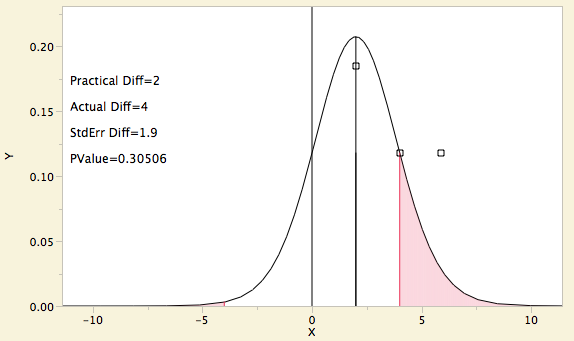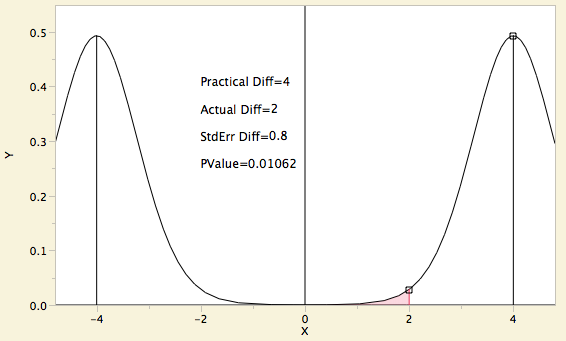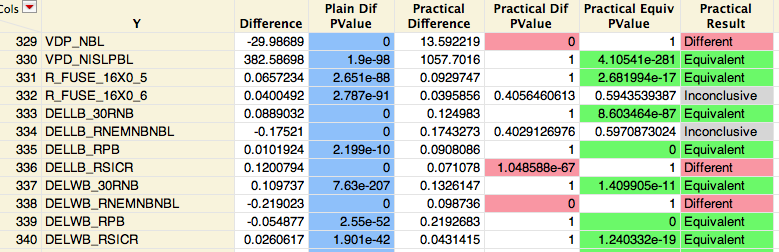Choose Language Hide Translation Bar
Big Data always has significant differences but not always practical differences: Practical significance and equivalence

When you have millions of observations of real data and do a simple fit across two variables, if you don’t get a significant test, then it is strong evidence of fraud. The one kind of data that is reliably non-significant for very tall data tables is simulated data. We live in a world with many subtle forces acting in many complex ways — so things are generally different by a little, even if they are not different by a lot.

In an age of Big Data, when we have millions of observations, we can expect almost all tests to be significant. But in most cases, we don’t care about small differences. What we want to know is if the difference is larger than what we care about.

In industry, we usually have a pretty good idea of what change we care about — we have specification limits, the Lower Spec Limit (LSL) and Upper Spec Limit (USL). If the difference is bigger than some fraction of that specification range, say 10%, then we know we care to look at it. Engineering data usually has specification limits to guide us on how sensitive to be to changes.

If we don’t have specification limits, we use something similar. Assuming the mean difference is small compared to the natural variation and that the process is reasonably behaved with a capability of 1, then we use 6*sigma as the spec range. We use IQR/(Q(.75)-Q(.25)) to estimate sigma, where Q is the normal quantile, and then we care about 10% of that interval.

If we have a practical difference in mind, then we can easily construct a hypothesis test that the absolute value of real difference is less than equal to the practical difference. This test will not always be significant if you have millions of observations — rather, it will tend to be either very significant or the opposite.

Here is a picture of how the test works. Suppose that the practical difference that you care about is 2, and the actual difference is 4. You construct a Student’s t distribution scaled by the standard error of the difference around the practical difference. Then you calculate the tail probability past the actual difference, which in this case is about .3. But that is just one direction. You also have to look at the negative of the actual difference and measure the probability in that tail of the same distribution. Now you have a p-value for the practical difference test. This is the test you should do to tell you about changes you care about.However, that test may be the reverse of the test that you really want. If you want reassurance that the differences are small, then it doesn’t make sense to use the lack of evidence that the difference is not big. You really want to test in the reverse way, to be fairly sure that the difference is smaller than the practical difference.

Tests in that direction are called equivalence tests. They are used most often in the regulated medicine and medical devices industries to prove that a change doesn’t affect the results. Equivalence tests should be taught to introductory statistics students so that they understand that accepting a null hypothesis does not prove the null — it just means you don’t have enough evidence to reject it.

The standard approach to equivalence tests is the TOST – two one-sided t-tests. You test that the difference is less than the practical difference and also you test that the difference is greater than the negative of the practical difference. Thus, you prove that the difference is smaller in absolute value than the difference.

Suppose that the difference you care about, the practical difference, is 4, and the actual difference is 2. To test that the difference is less than the practical difference, you put a Student’s t distribution with the appropriate degrees of freedom and scaled by the standard error of the difference over the practical difference, 4. Note that the area in the low tail at the actual difference is about .01. Now you put the Student’s t distribution over the negative of the practical difference, -4. You measure the area in the right tail, which here is very, very small. Then you take the maximum of the two p-values, which here turns out to be .01. Thus, you have demonstrated practical equivalence at the .01 significance level.Now we have both tools. So when we compare means, with reference to a practical difference, we have two p-values and three possible conclusions: Practically Equivalent, Practically Different and Practically Inconclusive, if both p-values are not significant.In the means comparisons above, all tests are extremely significant for testing that the mean is zero; these are the p-values shaded blue. But only three of the responses are practically different, i.e., significantly different more than the practical difference. These are the p-values shaded in red. Seven of the responses are practically equivalent – i.e., the difference is significantly less than the practical difference in absolute value. These are shaded in green. Two of the tests are inconclusive — the difference is significantly different than zero, but not significantly different compared to the practical difference, and not significantly smaller than the practical different (equivalent) either.

Now you can go through the comparisons and quickly identify how each of the many responses has changed its mean across the factor, even if you have millions of observations.

Practical difference tests are available in JMP 11 in the Response Screening platform, and Equivalence tests are available there as well as in Oneway and the new Nonlinear.

Note: This is part of a Big Statistics series of blog posts by John Sall. Read all of his Big Statistics posts.

Article Labels

There are no labels assigned to this post.

Article Tags
1 Comment
Visitor

Mike Clayton wrote:

These ideas should be elaborated in webinar format for high school STEM classes.

These are important concepts that need to be illustrated (as you have done) and are not well illustrated in many text books. Data rich as we are now days, the old hypothesis tests are much less useful at first glance, but you have salvaged the p-value for big data by use of practical limits and a novel (to me) visualization and table (when many people are now attacking p-values as dangerous or useless in many ways.)

Caveat: I am not a statistician.